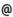## "Babes-Bolyai" University of Cluj-Napoca Faculty of Mathematics and Computer Science

 Biomathematics
 Code Semes-ter Hours: C+S+L Credits Type Section ME278 2 2+1+1 7 compulsory Matematică Aplicată
 Teaching Staff in Charge
 Prof. PRECUP Radu, Ph.D., r.precupmath.ubbcluj.ro
 Lect. BUICA Adriana, Ph.D., abuicamath.ubbcluj.ro
 Aims Main models from biology are presented.
 Content 1. Population dynamics: single species models; Lotka-Volterra type models; equilibrium solutions; stability; hysteresis. 2. Methods of nonlinear analysis: the fixed point theorems of Banach, Schauder and Leray-Schauder; methods of upper and lower solutions and monotone iteration principle; applications to a delay integral equation from biology. 3. Reaction-diffusion systems: conservation equation; reaction-diffusion mechanisms; Turing's theory on diffussion-driven instability; spatial pattern with reaction-diffusion mechanisms; applications in morphogenesis. 4. Mathematical models in epidemiology: SIR models; methods of nonlinear analysis for the treatment of nonlinear equations from biomathematics; geographic spread of epidemics; travelling wave solutions.
 References 1. DIEKMANN O., DURRETT R., HADELER K., MAINI P., SMITH H.L. (eds.), Mathematics inspired by biology, Springer, 1999. 2. LEUNG A.W., Systems of nonlinear partial differential equations. Applications to biology and engineering, Kluwer, 1989 3. BRAUER F., CASTILLO-CHAVEZ C., Mathematical models in population biology and epidemiology, Spinger, 2001 4. MURRAY J.D., Mathematical biology, Springer, 1987 5. PRECUP R., Lectii de ecuatii cu derivate partiale, Presa Universitara Clujeana, 2004 6. PRECUP R., Methods in nonlinear integral equations, Kluwer, 2002 7. RASS L., RADCLIFFE J., Spatial deterministic epidemics, AMS, 2003
 Assessment written examination